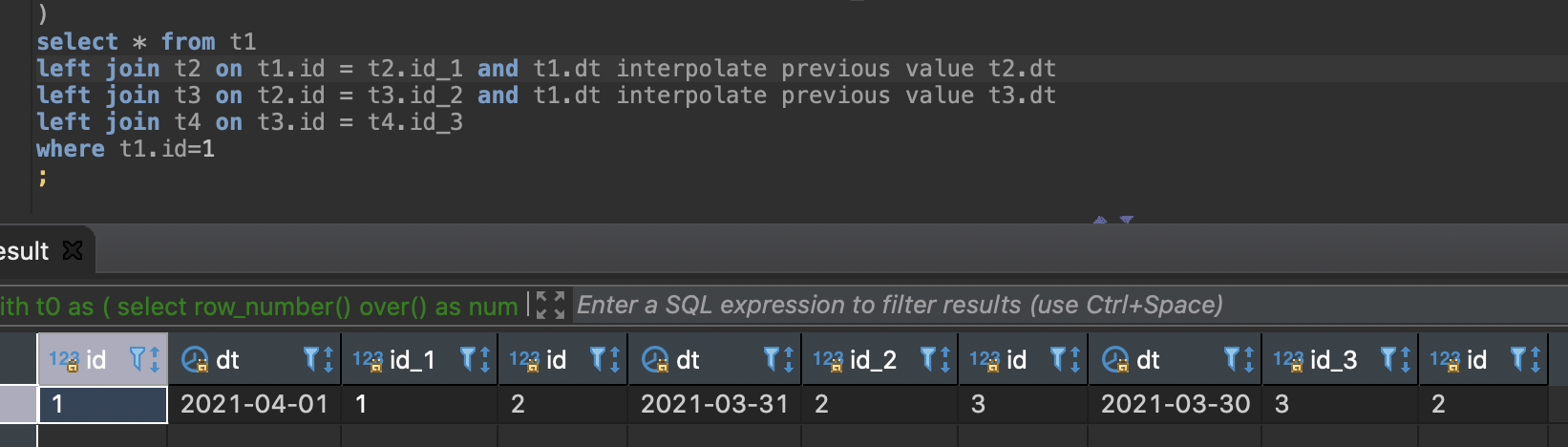# Different results for one query due to optimizer

edited April 2021

I have query like:

with
t0 as ( select row_number() over() as num from (select 1 from (select date(0) + interval '1 second' as sec union all select date(0) + interval '1000 seconds') _ timeseries tm as '1 second' over (order by sec) ) _ ),
t1 as ( select num as id, (current_date - num + 1) as dt from t0 ),
t2 as ( select num as id_1, num + 1 as id, (current_date - num) as dt from t0 ),
t3 as ( select num + 1 as id_2, num + 2 as id, (current_date - num - 1) as dt from t0 ),
t4 as ( select num + 2 as id_3, _.id as id from t0 cross join (select 1 as id union all select 2 union all select 3) _ )
select * from t1
left join t2 on t1.id = t2.id_1 and t1.dt interpolate previous value t2.dt
left join t3 on t2.id = t3.id_2 and t1.dt interpolate previous value t3.dt
left join t4 on t3.id = t4.id_3
where t1.id=1 ;

Optimizer makes the last left join earlier than the last join with interpolate previous value thats why I "lose" several rows received from t4.BUT
If I use hint SYNTACTIC_JOIN and join order is fixed, these rows exist.Is is expected behavior that the query result depends on join order from query plan designed by optimizer?

Tagged:

• It's not a expected behavior. Looks like a bug. What's the vertica version.

• It replayed on Vertica Analytic Database v9.2.1-5 (1 and more nodes) and v10.1.0-0 (1 node).

• Would it be possible to log a support case for this? We need to reproduce and log a jira for this. To reproduce this issue we need table definition for all the tables used in the query and some sample data.

• edited April 2021

You can use my initial query because all data is generated inside CTE.

``` with
t0 as ( select row_number() over() as num from (select 1 from (select date(0) + interval '1 second' as sec union all select date(0) + interval '1000 seconds') _ timeseries tm as '1 second' over (order by sec) ) _ ),
t1 as ( select num as id, (current_date - num + 1) as dt from t0 ),
t2 as ( select num as id_1, num + 1 as id, (current_date - num) as dt from t0 ),
t3 as ( select num + 1 as id_2, num + 2 as id, (current_date - num - 1) as dt from t0 ),
t4 as ( select num + 2 as id_3, _.id as id from t0 cross join (select 1 as id union all select 2 union all select 3) _ )
select * from t1
left join t2 on t1.id = t2.id_1 and t1.dt interpolate previous value t2.dt
left join t3 on t2.id = t3.id_2 and t1.dt interpolate previous value t3.dt
left join t4 on t3.id = t4.id_3
where t1.id=1 ;
```
``` with
t0 as ( select row_number() over() as num from (select 1 from (select date(0) + interval '1 second' as sec union all select date(0) + interval '1000 seconds') _ timeseries tm as '1 second' over (order by sec) ) _ ),
t1 as ( select num as id, (current_date - num + 1) as dt from t0 ),
t2 as ( select num as id_1, num + 1 as id, (current_date - num) as dt from t0 ),
t3 as ( select num + 1 as id_2, num + 2 as id, (current_date - num - 1) as dt from t0 ),
t4 as ( select num + 2 as id_3, _.id as id from t0 cross join (select 1 as id union all select 2 union all select 3) _ )
select /*+SYNTACTIC_JOIN*/ * from t1
left join t2 on t1.id = t2.id_1 and t1.dt interpolate previous value t2.dt
left join t3 on t2.id = t3.id_2 and t1.dt interpolate previous value t3.dt
left join t4 on t3.id = t4.id_3
where t1.id=1 ;
```

This query is result of simplification of real data sets and query.